# Charging a Capacitor

Whenever we connect an uncharged or partly charged capacitor with a voltage source whos voltage is more than the voltage of the capacitor (in case of partly charged capacitor) it recieves charge from the source and the voltage across the capacitor rises exponentially until it becomes equal and opposite to voltage of the source.

Let us connect one capacitor of capacitance C in series with a resistor of resistance R. We also connect this series combination of capacitor and resistor with a battery of voltage V through a push switch S.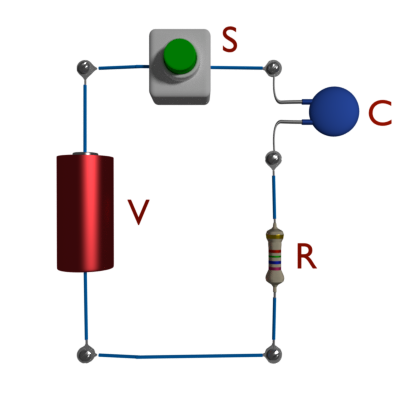Let us assume the capacitor is initially uncharged. When we push the switch, as the capacitor is uncharged, no voltage gets developed across the capacitor, thus the capacitor will behave as short circuit. At that moment the charge just starts accumulating in the capacitor. The current through the circuit will only be limited by resistance R.

So, the initial current is V/R. Now gradually the voltage is being developed across the capacitor, and this developed voltage is in the opposite of the polarity of the battery. As a result the current in the circuit gets gradually decreased. When the voltage across the capacitor becomes equal and opposite of the voltage of the battery, the current becomes zero. The voltage gradually increases across the capacitor during charging. Let us consider the rate of increase of voltage across the capacitor is dv/dt at any instant t. The current through the capacitor at that instant is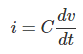Applying, Kirchhoff’s Voltage Law, in the circuit at that instant, we can write,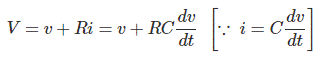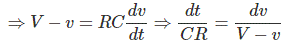Integrating both side we get,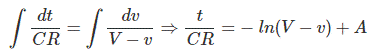Now, at the time of switching on the circuit, voltage across the capacitor was zero. That means, v = 0 at t = 0.
Putting these values in above equation, we getAfter getting the value of A, we can rewrite the above equation as,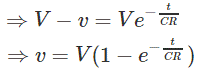Now, we know that,This is the expression of charging current I, during process of charging.
The current and voltage of the capacitor during charging is shown below.Here in the above figure, Io is the initial current of the capacitor when it was initially uncharged during switching on the circuit and Vo is the final voltage after the capacitor gets fully charged.
Putting t = RC in the expression of charging current (as derived above), we get,So at the time t = RC, the value of charging current becomes 36.7% of initial charging current (V / R = Io) when the capacitor was fully uncharged. This time is known as the time constant of the capacitive circuit with capacitance value C farad along with the resistance R ohms in series with the capacitor. The value of voltage developed across that capacitor at the time constant is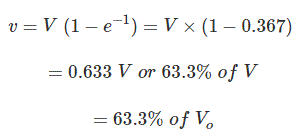Here Vo is the voltage finally developed across the capacitor after the capacitor is fully charged and it is same as source voltage (V = Vo).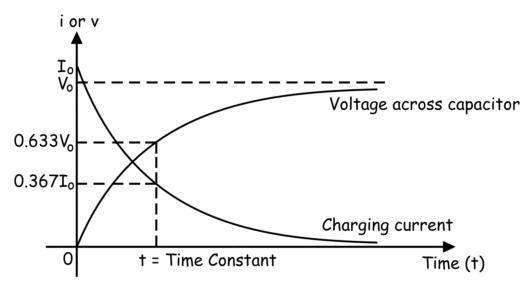Want To Learn Faster? 🎓
Get electrical articles delivered to your inbox every week.
No credit card required—it’s 100% free.